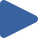# Quick Sort

## Animation, code, analysis, and discussion of quick sort on 4 initial conditions.

How to use: Press "Play all", or choose thebutton.

 Play All Play animationRandomPlay animationNearly SortedPlay animationReversedPlay animationFew Unique### ALGORITHM

``````_# choose pivot_
swap a[1,rand(1,n)]

_# 2-way partition_
k = 1
for i = 2:n, if a[i] < a, swap a[++k,i]
swap a[1,k]
_→ invariant: a[1..k-1] < a[k] <= a[k+1..n]_

_# recursive sorts_
sort a[1..k-1]
sort a[k+1,n]
``````

### DISCUSSION

When carefully implemented, quick sort is robust and has low overhead. When a stable sort is not needed, quick sort is an excellent general-purpose sort – although the 3-way partitioning version should always be used instead.

The 2-way partitioning code shown above is written for clarity rather than optimal performance; it exhibits poor locality, and, critically, exhibits O(n2) time when there are few unique keys. A more efficient and robust 2-way partitioning method is given in Quicksort is Optimal by Robert Sedgewick and Jon Bentley. The robust partitioning produces balanced recursion when there are many values equal to the pivot, yielding probabilistic guarantees of O(n·lg(n)) time and O(lg(n)) space for all inputs.

With both sub-sorts performed recursively, quick sort requires O(n) extra space for the recursion stack in the worst case when recursion is not balanced. This is exceedingly unlikely to occur, but it can be avoided by sorting the smaller sub-array recursively first; the second sub-array sort is a tail recursive call, which may be done with iteration instead. With this optimization, the algorithm uses O(lg(n)) extra space in the worst case.

### KEY

• Black values are sorted.
• Gray values are unsorted.
• Dark gray values denote the current interval.
• A pair of red triangles mark k and i (see the code).

### PROPERTIES

• Not stable
• O(lg(n)) extra space (see discussion)
• O(n2) time, but typically O(n·lg(n)) time